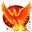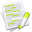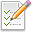wx.Colour¶

A colour is an object representing a combination of Red, Green, and Blue (RGB) intensity values and an Alpha value, and is used to determine drawing colours.

See the entry for wx.ColourDatabase for how a pointer to a predefined, named colour may be returned instead of creating a new colour.

Valid RGB values are in the range 0 to 255.

You can retrieve the current system colour settings with wx.SystemSettings.Class Hierarchy¶Inheritance diagram for class Colour:Methods Summary¶

 __init__ Default constructor. Alpha Returns the alpha value, on platforms where alpha is not yet supported, this always returns wx.ALPHA_OPAQUE. AlphaBlend Blend colour, taking alpha into account. Blue Returns the blue intensity. ChangeLightness wx.Colour wrapper for ChangeLightness(r,g,b,ialpha). Get Returns the RGB intensity values as a tuple, optionally the alpha value as well. GetAsString Converts this colour to a String using the given flags. GetIM Returns an immutable representation of the wx.Colour object, based on namedtuple. GetLuminance Return the perceived brightness of the colour. GetPixel GetRGB Gets the RGB or RGBA colour values as a single 32 bit value. GetRGBA Gets the RGB or RGBA colour values as a single 32 bit value. Green Returns the green intensity. IsOk Returns True if the colour object is valid (the colour has been initialised with RGB values). IsSolid Returns True if the color can be described using RGB values, i.e. MakeDisabled Make a disabled version of this colour. MakeGrey Create a grey colour from (in/out) rgb parameters using integer arithmetic. MakeMono Assigns the same value to r, g, b: 0 if on is false , 255 otherwise. Red Returns the red intensity. Set Sets the RGB intensity values using the given values (first overload), extracting them from the packed long (second overload), using the given string (third overload). SetRGB Sets the RGB or RGBA colour values from a single 32 bit value. SetRGBA Sets the RGB or RGBA colour values from a single 32 bit value. __bool__ __getitem__ __len__ __nonzero__ __reduce__ __repr__ __setitem__ __str__ _copyFrom For internal use only. __ne__ Tests the inequality of two colours by comparing individual red, green, blue intensities and alpha values. __eq__ Tests the equality of two colours by comparing individual red, green, blue intensities and alpha values.Properties Summary¶

 Pixel See GetPixel RGB See GetRGB and SetRGB RGBA See GetRGBA and SetRGBA alpha See Alpha blue See Blue green See Green red See RedClass API¶

class wx.Colour(Object)

Possible constructors:

Colour()

Colour(red, green, blue, alpha=ALPHA_OPAQUE)

Colour(colRGB)

Colour(colour)

A colour is an object representing a combination of Red, Green, and Blue (RGB) intensity values and an Alpha value, and is used to determine drawing colours.

Methods¶

__init__(self, *args, **kw)Overloaded Implementations:

__init__ (self)

Default constructor.

__init__ (self, red, green, blue, alpha=ALPHA_OPAQUE)

Parameters
• red (int) – The red value.

• green (int) – The green value.

• blue (int) – The blue value.

• alpha (int) – The alpha value. Alpha values range from 0 (wxwx.ALPHA_TRANSPARENT) to 255 (wxwx.ALPHA_OPAQUE).

__init__ (self, colRGB)

Parameters

colRGB (long) – A packed RGB value.

__init__ (self, colour)

Copy constructor.

Parameters

colour (wx.Colour) –

Alpha(self)

Returns the alpha value, on platforms where alpha is not yet supported, this always returns wx.ALPHA_OPAQUE.

Return type

int

static AlphaBlend(fg, bg, alpha)

Blend colour, taking alpha into account.

Parameters
• fg (int) –

• bg (int) –

• alpha (float) –

Return type

int

New in version 2.9.0.

Blue(self)

Returns the blue intensity.

Return type

int

ChangeLightness(self, *args, **kw)Overloaded Implementations:

ChangeLightness (self, ialpha)

wx.Colour wrapper for ChangeLightness(r,g,b,ialpha).

Parameters

ialpha (int) –

Return type

wx.Colour

New in version 2.9.0.

ChangeLightness (r, g, b, ialpha)

Utility function that simply darkens or lightens a color, based on the specified percentage ialpha.

ialpha of 0 would be make the color completely black, 200 completely white and 100 would not change the color.

Parameters
• r (int) –

• g (int) –

• b (int) –

• ialpha (int) –

Return type

tuple

Returns

( r, g, b )

New in version 2.9.0.

Get(self, includeAlpha=True)

Returns the RGB intensity values as a tuple, optionally the alpha value as well.

Return type

tuple

Returns

( r, g, b) or (r, g, b, a )

GetAsString(self, flags=C2S_NAME|C2S_CSS_SYNTAX)

Converts this colour to a String using the given flags.

The supported flags are C2S_NAME , to obtain the colour name (e.g. wx.Colour == “red”), C2S_CSS_SYNTAX , to obtain the colour in the “rgb(r,g,b)” or “rgba(r,g,b,a)” syntax (e.g. wx.Colour == “rgba(255,0,0,0.333)”), and C2S_HTML_SYNTAX , to obtain the colour as “#” followed by 6 hexadecimal digits (e.g. wx.Colour == “#FF0000”).

This function returns empty string if the colour is not initialized (see IsOk ). Otherwise, the returned string is always non-empty, but the function asserts if the colour has alpha channel (i.e. is non opaque) but C2S_CSS_SYNTAX (which is the only one supporting alpha) is not specified in flags.

Parameters

flags (long) –

Return type

string

New in version 2.7.0.

Note

For non-solid (i.e. non-RGB) colour this function returns “rgb(??, ?? ??)” or “#??????”.

GetIM(self)

Returns an immutable representation of the wx.Colour object, based on namedtuple.

This new object is hashable and can be used as a dictionary key, be added to sets, etc. It can be converted back into a real wx.Colour with a simple statement like this: obj = wx.Colour(imObj).

GetLuminance(self)

Return the perceived brightness of the colour.

This value is computed using the simple:

( 0.299*R + 0.587*G + 0.114*B )

formula with the coefficients taken from the RGB to YIQ conversion formula and R , G and B being the values of the corresponding colour channels normalized to 0..1 range, so that the return value is 0 for black and 1 for white.

Return type

float

New in version 4.1/wxWidgets-3.1.3.

GetPixel(self)
Return type

wx.IntPtr

GetRGB(self)

Gets the RGB or RGBA colour values as a single 32 bit value.

The returned value is of the same form as expected by SetRGB and SetRGBA .

Notice that GetRGB returns the value with 0 as its highest byte independently of the value actually returned by Alpha . So for a fully opaque colour, the return value of GetRGBA is 0xFFBBGGRR while that of GetRGB is 0x00BBGGRR .

Return type

wx.int

New in version 2.9.1.

GetRGBA(self)

Gets the RGB or RGBA colour values as a single 32 bit value.

The returned value is of the same form as expected by SetRGB and SetRGBA .

Notice that GetRGB returns the value with 0 as its highest byte independently of the value actually returned by Alpha . So for a fully opaque colour, the return value of GetRGBA is 0xFFBBGGRR while that of GetRGB is 0x00BBGGRR .

Return type

wx.int

New in version 2.9.1.

Green(self)

Returns the green intensity.

Return type

int

IsOk(self)

Returns True if the colour object is valid (the colour has been initialised with RGB values).

Return type

bool

IsSolid(self)

Returns True if the color can be described using RGB values, i.e.

is solid, False if it is a pattern (currently only possible on macOS)

Return type

bool

MakeDisabled(self, *args, **kw)Overloaded Implementations:

MakeDisabled (self, brightness=255)

Make a disabled version of this colour.

This method modifies the object in place and returns the object itself.

Parameters

brightness (int) –

Return type

wx.Colour

New in version 2.9.5.

MakeDisabled (r, g, b, brightness=255)

Create a disabled (dimmed) colour from (in/out) rgb parameters.

Parameters
• r (int) –

• g (int) –

• b (int) –

• brightness (int) –

Return type

tuple

Returns

( r, g, b )

New in version 2.9.0.

static MakeGrey(*args, **kw)Overloaded Implementations:

MakeGrey (r, g, b)

Create a grey colour from (in/out) rgb parameters using integer arithmetic.

Parameters
• r (int) –

• g (int) –

• b (int) –

Return type

tuple

Returns

( r, g, b )

New in version 2.9.0.

MakeGrey (r, g, b, weight_r, weight_g, weight_b)

Create a grey colour from (in/out) rgb parameters using floating point arithmetic.

Defaults to using the standard ITU-T BT.601 when converting to YUV, where every pixel equals (R weight_r) + (G weight_g) + (B weight_b).

Parameters
• r (int) –

• g (int) –

• b (int) –

• weight_r (float) –

• weight_g (float) –

• weight_b (float) –

Return type

tuple

Returns

( r, g, b )

New in version 2.9.0.

static MakeMono(on)

Assigns the same value to r, g, b: 0 if on is false , 255 otherwise.

Parameters

on (bool) –

Return type

tuple

Returns

( r, g, b )

New in version 2.9.0.

Red(self)

Returns the red intensity.

Return type

int

Set(self, *args, **kw)

Sets the RGB intensity values using the given values (first overload), extracting them from the packed long (second overload), using the given string (third overload).

When using third form, Set accepts: colour names (those listed in wx.ColourDatabase), the CSS-like "rgb(r,g,b)" or "rgba(r,g,b,a)" syntax (case insensitive) and the HTML-like syntax: "#" followed by 6 hexadecimal digits for red, green, blue components.

Returns True if the conversion was successful, False otherwise.

New in version 2.7.0.Overloaded Implementations:

Set (self, red, green, blue, alpha=ALPHA_OPAQUE)

Parameters
• red (int) –

• green (int) –

• blue (int) –

• alpha (int) –

Set (self, RGB)

Parameters

RGB (long) –

Set (self, str)

Parameters

str (string) –

Return type

bool

SetRGB(self, colRGB)

Sets the RGB or RGBA colour values from a single 32 bit value.

The arguments colRGB and colRGBA should be of the form 0x00BBGGRR and 0xAABBGGRR respectively where 0xRR , 0xGG , 0xBB and 0xAA are the values of the red, green, blue and alpha components.

Notice the right-to-left order of components!

Parameters

colRGB (wx.int) –

New in version 2.9.1.

SetRGBA(self, colRGBA)

Sets the RGB or RGBA colour values from a single 32 bit value.

The arguments colRGB and colRGBA should be of the form 0x00BBGGRR and 0xAABBGGRR respectively where 0xRR , 0xGG , 0xBB and 0xAA are the values of the red, green, blue and alpha components.

Notice the right-to-left order of components!

Parameters

colRGBA (wx.int) –

New in version 2.9.1.

__bool__(self)
Return type

int

__getitem__(self, idx)

__len__(self)

__nonzero__(self)
Return type

int

__reduce__(self)

__repr__(self)

__setitem__(self, idx, val)

__str__(self)

_copyFrom(self, other)

For internal use only.

__ne__(self)

Tests the inequality of two colours by comparing individual red, green, blue intensities and alpha values.

Parameters

colour (wx.Colour) –

__eq__(self)

Tests the equality of two colours by comparing individual red, green, blue intensities and alpha values.

Parameters

colour (wx.Colour) –

Properties¶

Pixel

See GetPixel

RGB

See GetRGB and SetRGB

RGBA

See GetRGBA and SetRGBA

alpha

See Alpha

blue

See Blue

green

See Green

red

See Red